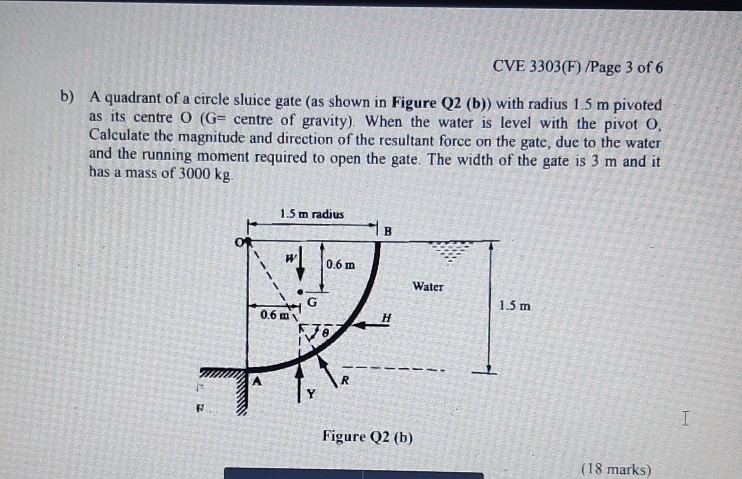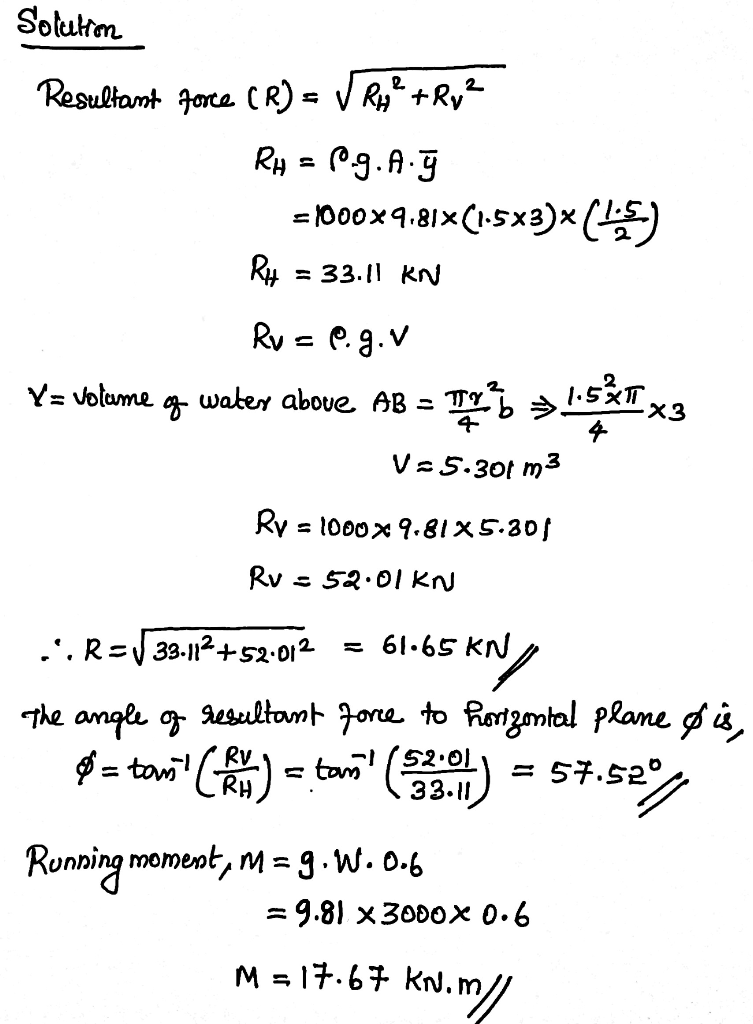# CVE 3303F) /Page 3 of 6 b) A quadrant of a circle sluice gate (as shown in Figure Q2 (b) with radius 1.5 m pivoted as its centre O (G centre of gravity). When the water is level with the pivot...CVE 3303F) /Page 3 of 6 b) A quadrant of a circle sluice gate (as shown in Figure Q2 (b) with radius 1.5 m pivoted as its centre O (G centre of gravity). When the water is level with the pivot O Calculate the magnitude and dircction of the resultant force on the gate, due to the water and the running moment required to open the gate. The width of the gate is 3 m and it has a mass of 3000 kg. 1.5 m radius W 0.6m Water 1.5 m 0.6m Figure Q2 (b) (18 marks)In case of any query please comment. If you have liked the solution then please THUMBS UP.In the crest gate in a dam shown, surface AB forms the arc of a circle of 3 m radius subtending 5 at the hinge. The gate is 3 m long with water surface at B. Compute the amount and location of the horizontal and vertical components of the total hydrostatic force on AB. Determine also the amount and direction of their resultant. 2. 2 m 3 In the crest gate in a dam shown, surface AB forms the arc...